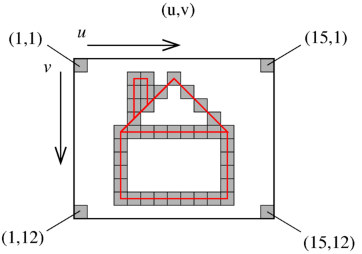Warning

## Image coordinate system

The choice of an image coordinate system is a matter of convention in most cases. As introduced in the lectures, we will use the left handed image coordinate system, with the first axis (u) pointing right and the second axis (v) pointing down. Coordinates of a point are then written as an algebraic column vector (euclidean) <latex>(u, v)^\top</latex>. In Matlab, we will write e.g.

[u;v]

Since 1-based indexing is used in Matlab, the centre of the top left pixel has coordinates [1;1] and the centre of the bottom right pixel has coordinates [count_u; count_v ], where count_u is number of image columns and count_v is number of image rows (both in pixels). See figure 1.Fig. 1: Image coordinate system

When drawing points or lines in Matlab, correct orientation of ordinate must be set.

plot( u, v );
set( gca, 'ydir', 'reverse' );

It is also possible to draw a bitmap image first, and then draw points or lines over the bitmap. Correct orientation is then set automatically by imaging the bitmap.

im = imread( 'image.jpg' );
image( im );
hold on
plot( u, v );
hold off

Matlab considers images as ordinary matrices, so the standard matrix indexation (row, column) is used. Let [u;v] be the integer coordinates in the grayscale image (centre of a pixel). Then the intensity of the pixel is obtained as

im(v,u)

## Matlab Help for Typical Situations in Homeworks

#### Some recommendations for the fastest progress

1. Do not trust any results unless you verified them.
3. Try to visualize your results
4. Each Matlab command has help. E.g. for plot
help plot % text version
doc plot % html version
5. Try to encapsulate general tasks into a functions and reuse them.
6. Remind the rule 1.
##### Debugging

Matlab has a powerful debugger. Either put breakpoints everywhere you want, or use the command keyboard in your code to stop processing at the place. Exit the debugging mode:

dbquit

You can also start debugging automatically in case of error by turning on

dbstop error

#### Vectors and its transformations

Vectors are stored as column matrices.

u = [1;2];
X = [1;2;3];
Xmore = [1 2 3; 4 5 6]';  % transpose at the end

Vectors are multiplied by a matrices on the left:

P = [1 0 0 -5; 0 1 0 -6; 0 0 1 1];  % a camera
ux_homog = P * [X;1]; % projection

#### Special matrices: Identity, Zeros, Ones

I = eye(3); % 3x3 identity
I2 = diag( [1 1 1] ); % same result
Z = zeros(3); % 3x3 matrix of zeroes
O = ones(3); % 3x3 matrix of ones

#### Euclidean distance of two 3D points

X1 = [1;2;3];
X2 = [4;5;6];
d2 = norm(X1-X2); % cannot be used for more vectors
d2 = sqrt(sum((X1-X2).^2)); % for matrices with points stored in columns

#### Dot (scalar) product

u1 = [4;5;1];
u2 = [7;8;1];
dot12 = u1' * u2;

#### Cross product

u1 = [4;5;1];
u2 = [7;8;1];
u12x = cross(u1,u2);
% verification of orthogonality by dot product
% (remember the recommendations 1 and 2)
u12x' * u1 % should be zero
u12x' * u2 % should be zero